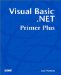# Operator Precedence

Until this point, we have covered the arithmetic, relational, and logical operators that Visual Basic .NET makes available. In complex expressions, you might use all three operators in a single program statement. How does Visual Basic .NET decide how to process each expression in such complex statements? Once again, operator precedence determines the sequence that Visual Basic .NET will follow.

Table 10.6 presents the order for all of Visual Basic .NET's operators, starting with the highest precedence and working down to the lowest precedence. (Note that Table 10.6 includes a few operators that are covered in later chapters.)

##### Table 10.6. Visual Basic .NET Operator Precedence

Operator

Name

Type

^

Exponentiation

Arithmetic

-

Negation

Arithmetic

* , /

Multiplication, division

Arithmetic

\

Integer division

Arithmetic

Mod

Modulus arithmetic

Arithmetic

+ , -

Arithmetic

&

String concatenation

String concatenation

=

Equality

Relational

`  <>  `

Inequality

Relational

< , >

Less than, greater than

Relational

`  >=  `

Greater than or equal to

Relational

`  <=  `

Less than or equal to

Relational

Like

String comparison

String comparison

`  Is  `

Object comparison

Object comparison

TypeOf...Is

Type comparison

Type comparison

Not

Negation

Logical

And , AndAlso

And, AndAlso

Logical

Or , Xor , OrElse

Or , Exclusive Or , OrElse

Logical

=

Assignment

Assignment

As a general rule, the arithmetic operators have highest precedence, followed by the relational operators, followed by the logical operators. You might want to dog-ear this page because you will probably need to refer to this table later on, when the program examples become a little more complex. You should keep in mind that you can override the operator precedence by using parentheses to force the order in which expressions are evaluated (refer to Chapter 9).Visual Basic .NET Primer Plus
ISBN: 0672324857
EAN: 2147483647
Year: 2003
Pages: 238
Authors: Jack Purdum

Similar book on Amazon